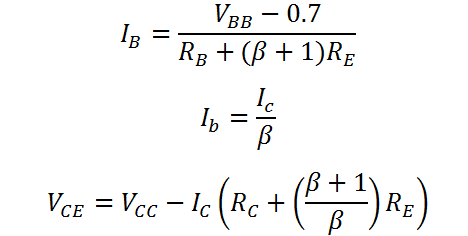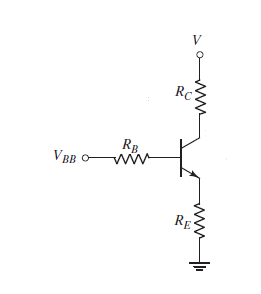# DC Analysis of a CE/CC BJT Amplifier with an Emitter Resistance

This CalcTown calculator calculates DC parameters for a BJT Common Emitter (CE) / Common Collector (CC) circuit with an emitter resistance. Since, the biasing schemes for a Common Base (CB) circuit is different, they are formulated in a separate calculator.

V
V
K-Ohm
K-Ohm
K-Ohm

#### Result

mA
mA
VClick here to view image

where

β=Common Emitter Current gain

VBE=0.7 V

VCC=Collector Biasing Voltage

and the resistances are marked in the diagram